# AP Board 8th Class Maths Solutions Chapter 1 Rational Numbers Ex 1.2

AP State Syllabus AP Board 8th Class Maths Solutions Chapter 1 Rational Numbers Ex 1.2 Textbook Questions and Answers.

## AP State Syllabus 8th Class Maths Solutions 1st Lesson Rational Numbers Exercise 1.2Question 1.
Represent these numbers on the number line.
(i) $$\frac{9}{7}$$
(ii) $$-\frac{7}{5}$$
Solution:
(i) $$\frac{9}{7}$$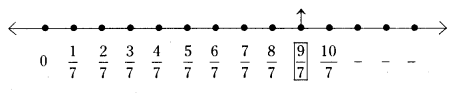(ii) $$-\frac{7}{5}$$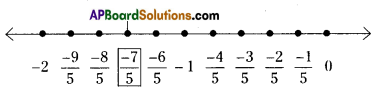Question 2.
Represent $$-\frac{2}{13}, \frac{5}{13}, \frac{-9}{13}$$ on the number line.
Solution: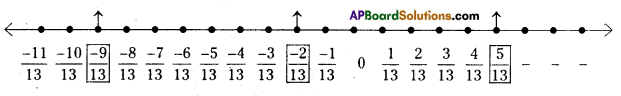Question 3.
Write five rational numbers which are smaller than $$\frac{5}{6}$$
Solution:
The rational number which are less than
$$\frac{5}{6}=\left\{\frac{4}{6}, \frac{3}{6}, \frac{2}{6}, \frac{1}{6}, \frac{0}{6}, \frac{-1}{6}, \frac{-2}{6} \ldots \ldots .\right\}$$

Question 4.
Find 12 rational numbers between -1 and 2.
Solution:

Question 5.
Find a rational number between $$\frac{2}{3}$$ and $$\frac{3}{4}$$
[Hint : First write the rational numbers with equal denominators.]
Solution:
The given rational numbers are $$\frac{2}{3}$$ and $$\frac{3}{4}$$
$$\frac{2}{3} \times \frac{4}{4}=\frac{8}{12}, \frac{3}{4} \times \frac{3}{3}=\frac{9}{12}$$
The rational numbers between $$\frac{8}{12}, \frac{9}{12}$$ is
$$\frac{\left(\frac{8}{12}+\frac{9}{12}\right)}{2}=\frac{\frac{17}{12}}{2}=\frac{17}{24}$$
(∵ the rational number between a, b is $$\frac{a+b}{2}$$ )
∴ the rational number between $$\frac{2}{3}$$ and $$\frac{3}{4}$$ is $$\frac{17}{24}$$Question 6.
Find ten rational numbers between $$-\frac{3}{4}$$ and $$\frac{5}{6}$$
Solution: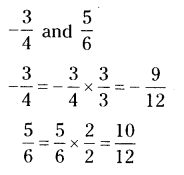The 10 rational numbers between $$-\frac{9}{12}$$ and $$\frac{10}{12}$$ are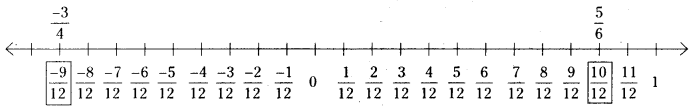∴ We can select any 10 rational numbers from the above number line.